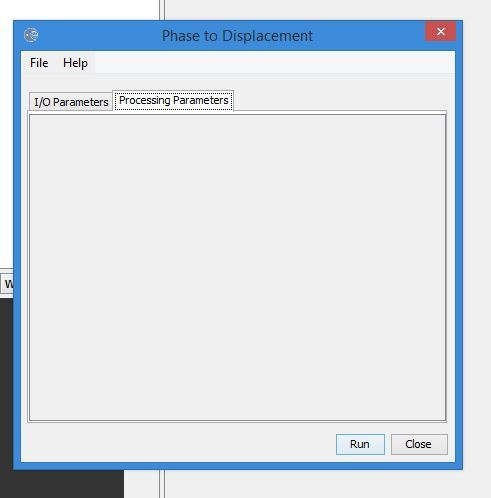# Difference between vertical and LOS displacement

The S-1 center frequency is 5405MHz (see abstracted metadata), you can easily calculate the wavelength from this as explained aboveI obtained 0.055 meter but i want to see it in the article or conference paper to give reference.

Here’s a reference for the 5.405GHz Centre frequency:

Sorry for rising up this post, but I checked with both formulas above. The displacement is fine, but with the vertical one calculated with formula provided (unwrapped phase * wavelength) / (-4 * PI * rad(cos(incidence angle)) )the result is not as expected (see below). The incidence angle is taken from the tie-point grid band incident_angle.Any suggestions?

24h latter - resolved using the average of the LIA from incident_angle band .

In SNAP after clicking menu item ‘radar>interferometric>unwrapping>snaphuImport’ there will be a box with 4 tabs ‘read’, ‘read unwrapped phase’, snaphuImport’ and ‘write’. But in GraphBuilder’s window after adding the same item as an icon there are only 2 tabs, ‘read’ and ‘snaphuImport’. I can add a ‘write’ tab but can not find the item corresponding to ‘read unwrapped phase’ tab. Is it available in SNAP?

the solution with the graph builder is obsolete. If was from a time when the import wasn’t as far developed.
You can just use “Snaphu Import”

hi mengdahl, i am new here,sorry to interrupt, i want to know where i can find the local incidence angle? thanks before

if your data was processed correctly, you find it under tie-point-grids (can also be used in the band maths):

1 Like

hi Abraun, we meet again, thanks! fyi my LOS DinSAR show in meter units, how if i want to change in cm units?

calculate in meters and then multiply with 1001 Like

ohhaha, well forget for the easiest steptbh i used the ‘phase to displacement’ at my first try, the processing parameters window didn’t show anything, is there any alternative?

yes, you can apply this formula manually in the band maths:

1 Likewait, i’m sorry, i still confused between LOS and vertical displacement formula. to calculate LOS in band math is (Unw_Phase * wavelength in mm) / (-4 * PI * cos(rad(incident_angle))) ? if i’m not wrong, that is vertical displacement formula right?

you are right, the cosine of the incidence angle is the LOS to vertical displacement factor.

so the LOS formula is unwrapped phase * wavelength/ -4*PI ? if the wavelength in cm, and then i want to calculate LOS to vertical displacement, should i use cm unit too?

I’m not sure, to be honest.

maybe i should do trial and error. well, thanks for your time to answer my questions, have a good day!

Since you say “not sure”, can you find an expert to describe the equation? Because (1) In optics the incident angle of a light line to a surface is between the “line of sight( LOS )” direction and the normal direction of the surface. If this is the same definition for the radio wave of InSAR, the ‘cos’ term in the equation zealandia_sarah shows above should be in the numerator to convert the LOSD to vertical displacement. (2) In my image processing by SNAP the result of vertical displacement looks too large( ~30cm ) on a region where there is no big geological event in the 12 days interval( S1A images ). Can you have some comments on this?

hello LiniC,

1. Incidence angle is the angle defined by the incident radar beam and the vertical (normal) to the intercepting surface as shown below.2. In terms of vertical displacement, as it is given by Z.Lu and D.Dzurisin, 2014 is as follows:
Let’s say we have two pixels, m and n which corresponds to two targets on the ground, the SAR phase for these points can be defined as follows:
phase_m = (-4pi/wavelength)*rm and phase_n = (-4pi/wavelength)*rn (where rn and rm are the distance between the satellite and the points on the ground.)

A second SAR image is acquired for the same area (let’s assume that the target point n has moved up by an amount of h). Now the phase for the point n’ which has moved can be expressed as follows:
phase_n’ = (-4pi/wavelength)(rn - hcos*theta) (where h is the vertical displacement of target n)

Hence, we form the interferogram by subtracting the phases of the two SAR images and the vertical displacement between the two points can be expressed as follows:
phase_n’ = (-4pi / wavelength)(hcos*theta)
So, in order to find the vertical displacement, we need to get the `cos` of the incidence angle

7 Likes